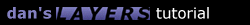2.2 Hovering

Hovering a layer up and down (or left and right) uses the same type of math as the circle. But this time we only have one direction to worry about it so it ends up being a little simpler.

```function hover() {
HovLayername = "layer1";  // name of the layer you want to move
HovAmplitude = 75;        // how far to move in any one direction
HovAngleinc = 2;          // incrementation in degrees
HovAnglestart = 0;        // angle to start at (use 180 to go down or left first)
HovDirection = "v";       // "v" for vertical, "h" for horizontal
HovSpeed = 10;            // speed of repetition
HovAngle = HovAnglestart;
HovLeft = document.layers[HovLayername].left - HovAmplitude*Math.sin(HovAnglestart*Math.PI/180);
HovTop = document.layers[HovLayername].top + HovAmplitude*Math.sin(HovAnglestart*Math.PI/180);
slidehover();
}

function slidehover() {
if (HovDirection == "h") {
var x = HovLeft + HovAmplitude*Math.sin(HovAngle*Math.PI/180);
document.layers[HovLayername].left = x;
}
if (HovDirection == "v") {
var y = HovTop - HovAmplitude*Math.sin(HovAngle*Math.PI/180);
document.layers[HovLayername].top = y;
}
HovAngle += HovAngleinc;
setTimeout("slidehover()",HovSpeed);
}
```1.1 Introduction 1.2 Overlapping 1.3 Nesting 1.4 Using JavaScript 2.1 Sliding Layers 2.2 Pre-Built Functions 2.3 Clipping Layers 2.4 Looping Animations 2.5 Changing Images 3.1 Mouse-Click Animation 3.2 Capturing Keystrokes 3.3 Drag and Drop 4.1 Making Demos 4.4 Problems 4.5 Screen Sizes Copyright © 1997 Dan Steinman. All rights reserved.